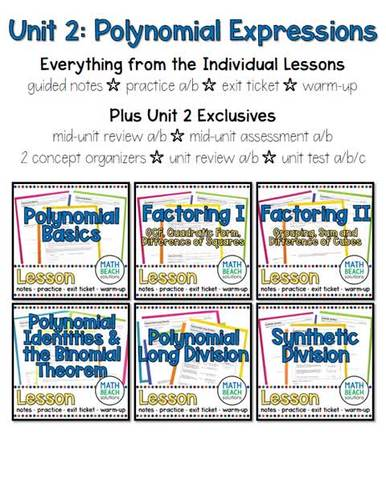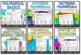# Unit 2: Polynomial Expressions - Algebra 2 CurriculumSubject
Resource Type
File Type

Zip

(51 MB|79 pages + keys)
Product Rating
Standards
Also included in:
1. This unit and activities bundle builds on students' knowledge of factoring quadratic expressions by introducing factoring polynomials of degree 3 and 4 using the following methods: grouping, sum and difference of cubes, quadratic form, and difference of squares. Polynomial operations including addin
\$40.00
\$25.00
Save \$15.00
• Product Description
• StandardsNEW

This unit builds on students' knowledge of factoring quadratic expressions by introducing factoring polynomials of degree 3 and 4 using the following methods: grouping, sum and difference of cubes, quadratic form, and difference of squares.

Polynomial operations including adding, subtracting, and multiplying polynomials is also covered. Students will use these techniques to prove polynomial identities. Students will use Pascal's triangle and the Binomial Theorem to expand expressions of the form (a + b)^n.

Finally, students will divide polynomials of degree 3 and 4 by linear and quadratic polynomials using polynomial long division and synthetic division.

Most resources are non-editable .pdf format.

Editable assessments are included for easy customization to your students' needs!

Editable versions of the mid-unit assessment and test are included in .docx format for MS Word.

UNIT 2 INCLUDES THE FOLLOWING LESSONS:

Lesson 1: Polynomial Basics

Students will:

• add, subtract, and multiply polynomial expressions

Lesson 2: Factoring I

Students will:

• factor polynomial expressions (up to degree 4)
• identify greatest common factors
• factor using the difference of squares factoring pattern
• factor using quadratic form trinomials factoring pattern

Lesson 3: Factoring II

Students will:

• factor polynomial expressions (up to degree 4)
• factor using the sum and difference of cubes factoring pattern
• factor by grouping

Lesson 4: Polynomial Identities and the Binomial Theorem

Students will:

• verify polynomial identities
• expand binomial expressions of the form (a+b)^n using the Binomial Theorem and Pascal’s triangle

Lesson 5: Polynomial Long Division

Students will:

• divide cubic and quartic polynomials by linear and quadratic polynomials using polynomial long division

Lesson 6: Synthetic Division

Students will:

• divide cubic and quartic polynomials by polynomials of the form using synthetic division

EACH LESSON CONTAINS THE FOLLOWING ELEMENTS:

All resources are in PDF format. Check out the preview for a detailed look!

• The Lesson Notes are designed for use as a note-taking guide combined with guided practice.

• Practice A and Practice B are suitable for use as in-class practice and homework. Two comparable forms are included for increased versatility.

• The Exit Ticket is designed for a quick concept check at the end of the class period.

• The Warm-Up is designed for next-day use to reinforce the previous day’s learning.

• One-page display versions of the exit ticket and warm-up are included. Display the PDF page on a screen for student viewing and have students record their responses on notebook paper or scratch paper to save on printing.

ADDITIONAL RESOURCES EXCLUSIVELY AVAILABLE IN UNIT 2 INCLUDE:

• 2 Concept Organizers offer complimentary alternatives for note-taking or post-lesson concept reinforcement.

• The Mid-Unit Review (Forms A and B) provides an opportunity for students to practice the concepts they learned in the first part of the unit.

• The Mid-Unit Assessment (Forms A and B) may be used as a test, quiz, or daily assignment to meet the specific needs of your students. Editable versions of Forms A and B are included as a .docx file for MS Word.

• The Unit Review (Forms A and B) helps students focus on the key concepts studied throughout the unit.

• The Unit Test (Forms A, B, and C) comes in two comparable multiple-choice forms (A and B) and one free-response form (C). The test key includes TEKS alignment for each question to facilitate easy data analysis. Editable versions of Forms A and B are included as a .docx file for MS Word.

STANDARDS:

A.SSE.2, A.APR.1, A.APR.2, A.APR.4, A.APR.5, A.APR.6 from the Common Core State Standards (CCSS)

A note from Allison about standards alignment: This resource was designed to provide ready-to-implement resources for teachers to use to facilitate their students’ mastery of the Common Core State Standards. A considerable effort has been made to ensure the standards are addressed thoroughly, but these resources will reflect my understanding of the standards. As always, continue to use your professional judgment and experience when lesson planning.

Rewrite simple rational expressions in different forms; write 𝘢(𝘹)/𝘣(𝘹) in the form 𝘲(𝘹) + 𝘳(𝘹)/𝘣(𝘹), where 𝘢(𝘹), 𝘣(𝘹), 𝘲(𝘹), and 𝘳(𝘹) are polynomials with the degree of 𝘳(𝘹) less than the degree of 𝘣(𝘹), using inspection, long division, or, for the more complicated examples, a computer algebra system.
Know and apply the Binomial Theorem for the expansion of (𝘹 + 𝘺)ⁿ in powers of 𝘹 and y for a positive integer 𝘯, where 𝘹 and 𝘺 are any numbers, with coefficients determined for example by Pascal’s Triangle.
Prove polynomial identities and use them to describe numerical relationships. For example, the polynomial identity (𝘹² + 𝘺²)² = (𝘹² – 𝘺²)² + (2𝘹𝘺)² can be used to generate Pythagorean triples.
Know and apply the Remainder Theorem: For a polynomial 𝘱(𝘹) and a number 𝘢, the remainder on division by 𝘹 – 𝘢 is 𝘱(𝘢), so 𝘱(𝘢) = 0 if and only if (𝘹 – 𝘢) is a factor of 𝘱(𝘹).
Understand that polynomials form a system analogous to the integers, namely, they are closed under the operations of addition, subtraction, and multiplication; add, subtract, and multiply polynomials.
Total Pages
79 pages + keys
Included
Teaching Duration
N/A
Report this Resource to TpT
Reported resources will be reviewed by our team. Report this resource to let us know if this resource violates TpT’s content guidelines.